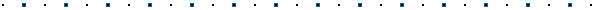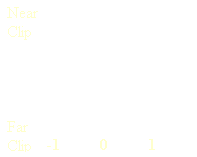#Projecting a Ray from 2D Screen Coordinates Written by Robert Dunlop Microsoft DirectX MVPNote: Code in this article was written for use with DirectX 7 under VC++ 6.0, and utilizes functions from the D3DMATH portion of the D3D framework included with the DX7 SDK.  Some modification may be required for use in other development environments.Update!  A sample demonstrating picking of meshes using DirectX 8 is now available
Go To: Improved Ray Picking

 Related Articles of Interest: View Oriented Billboards Improved Ray Picking## Introduction

Direct3D provides the means to project your 3D world onto the screen, but often 3D titles require the ability to convert screen coordinates into 3D, and to determine the objects that are visible at a location in the viewport.  Such techniques are often used for picking targets or plotting weapon trajectories in 3D games.

This article lies the initial groundwork required for establishing a ray pick routine, by providing algorithms and working code to convert a pixel coordinate into a ray in world space.## Overview of Ray Casting

The process of converting screen coordinates to 3D requires that we run through the vertex transformation process in reverse.  Basically, this is a five  step process:Convert screen coordinates, in pixels, to normalized coordinates, with an origin at the center of the viewport and values on each axis ranging from -1.0 to 1.0.Scale the normalized screen coordinates to the field of view.  The X and Y values attained will define the slope of the ray away from the center of the frustum in relation to depth.Calculate two points on the line that correspond to the near and far clipping planes.  These will be expressed in 3D coordinates in view space.Create a matrix that expresses an inverse of the current view transformation.Multiply these coordinates with the inverse matrix to transform them into world space.## Normalizing Screen Coordinates

We begin our journey with screen coordinates, corresponding to a pixel on the screen.  For the sake of a standard for this discussion, we will assume that coordinates are based on a 640 x 480 resolution.

To make use of these coordinates, we must first re-define them in terms of the visible area of the viewing frustum.  There are two differences that we must account for to do this:

1. The viewing frustum has an origin (0,0) at the center of the screen, while screen coordinates have an origin at the upper left of the screen.
2. Screen coordinates are expressed in number of pixels, while coordinates in the frustum are normalized to a range of -1.0 to 1.0.

To deal with this, we scale the incoming coordinates and offset them to the center.  We must also handle the difference in width and height, to compensate for the aspect ratio of the display:

#define WIDTH           640.0f
#define HEIGHT          480.0f
#define WIDTH_DIV_2      (WIDTH*0.5f)
#define HEIGHT_DIV_2     (HEIGHT*0.5f)
#define ASPECT            1.3333f

dx=(x/WIDTH_DIV_2-1.0f)/ASPECT;
dy=1.0f-y/HEIGHT_DIV_2;## Scaling Coordinates to the Frustum

The next step we must accomplish is to determine what these coordinates mean in view space.  To understand this, we must first take a brief look at the characteristics of the viewing frustum.

If you look at the frustum from any side, you can view it as a two dimensional triangle - we will use this perspective to analyze the problem, as it allows us to deal with one axis at a time.  In fact, the horizontal and vertical axis are not inter-related in this problem, so this part of the process really becomes a 2D problem.Imagine looking down on the frustum (viewing a triangle stretching away from the viewer), and dividing the triangle down the middle to form two equal triangles, as shown on the left.  This forms a pair of right triangles, which is useful to us because the ratios between sides of a right triangle are easily determined.  All we need to know is one angle (in addition to the 90 degree corner) and one side, and we can then determine all of the lengths and angles that make up the triangle.

Since we defined the frustum, we know the angle at which the sides of the frustum meet - this is the field of view we used to create the projection matrix in the first place.  Since we have split the frustum into two halves, each triangle has an angle at the origin of view equal to FOV/2.  If we calculate the tangent of this angle, we now have a value that corresponds to the ratio between the displacement on the X axis versus the distance away from the viewer on the Y.So, what does this do for us?  Well, now let's picture our viewing frustum in relation to our two clipping planes.  The clipping planes are at a know distance on the Z axis.  Since we know the ratio between X and Z (or Y and Z), we can determine the width of the frustum at a given depth.  The distance to the center of the frustum to the sides of the frustum (1 or -1) at a given depth is dist = Z * tan ( FOV / 2 )

With this information in hand, we can now calculate 3D coordinates in view space.  Since we know that the center of the screen corresponds to (0,0,Z), and since we know where the edge of the screen is, we can now interpolate any point in between.  Since we have already normalized our screen values, all we need to do is multiply them by the tangent to find a ratio for this point in the frustum.  We can adapt the previous lines of code to include this:

dx=tanf(FOV*0.5f)*(x/WIDTH_DIV_2-1.0f)/ASPECT;
dy=tanf(FOV*0.5f)*(1.0f-y/HEIGHT_DIV_2);

Note that this code calculates the tangent each time - this is only for clarity.  You can calculate this once at the start of the application, or use a constant if your projection will not change.

## Calculating the End Points of the Ray

Next, we can calculate the coordinates of the ray relative to the view, using end points at the near and far clipping planes:

p1=D3DVECTOR(dx*NEAR,dy*NEAR,NEAR);
p2=D3DVECTOR(dx*FAR,dy*FAR,FAR);

## Generating an Inverse of the View Matrix

To transform our coordinates back to world space, we will need an "inverse matrix" of our view matrix - that is, a matrix that does the reverse of the view matrix, and thus given a coordinate that has been multiplied by the view matrix, will return the original coordinate.

Fortunately, there is a handy helper function in the D3DMATH.CPP and D3DMATH.H files of the Direct3D framework that takes care of it for us:

lpDevice->GetTransform(D3DTRANSFORMSTATE_VIEW,&viewMatrix);
D3DMath_MatrixInvert(invMatrix,viewMatrix);

Note that not all matrixes can be inverted, but in normal use this should not be a problem.  It is stipulated, however, that the above function will fail if the last column of the matrix is not 0,0,0,1.

## Converting the Ray to World Coordinates

Finally, all that remains is to multiply these vectors by our inverse matrix, and there it is!  We have defined a line in 3D World coordinates that corresponds to the screen coordinates we started with.

D3DMath_VectorMatrixMultiply(p1,p1,invMatrix);
D3DMath_VectorMatrixMultiply(p2,p2,invMatrix);

## Working Code Sample for Ray Projection

To make it easier to grab, here is a complete copy of the source code presented above :

```#define	NEAR			10.0f
#define FAR			4000.0f
#define	FOV			0.8f
#define	WIDTH			640.0f
#define	HEIGHT			480.0f
#define	WIDTH_DIV_2		(WIDTH*0.5f)
#define	HEIGHT_DIV_2		(HEIGHT*0.5f)
#define ASPECT			1.3333f```
```void calcRay(int x,int y,D3DVECTOR &p1,D3DVECTOR &p2)
{
float dx,dy;
D3DMATRIX invMatrix,viewMatrix;

dx=tanf(FOV*0.5f)*(x/WIDTH_DIV_2-1.0f)/ASPECT;
dy=tanf(FOV*0.5f)*(1.0f-y/HEIGHT_DIV_2);
lpDevice->GetTransform(D3DTRANSFORMSTATE_VIEW,&viewMatrix);
D3DMath_MatrixInvert(invMatrix,viewMatrix);
p1=D3DVECTOR(dx*NEAR,dy*NEAR,NEAR);
p2=D3DVECTOR(dx*FAR,dy*FAR,FAR);
D3DMath_VectorMatrixMultiply(p1,p1,invMatrix);
D3DMath_VectorMatrixMultiply(p2,p2,invMatrix);
}```

## Where to Go from Here

If you are using this to retrieve a vector - for example, setting the direction for a projectile to travel - then at this point, you have what you need.  You can subtract the two vectors returned from the above function, and normalize them to get a vector representing direction in world space:

```D3DVECTOR calcDir(int x,int y)
{
D3DVECTOR p1,p2;```
```	calcRay(x,y,p1,p2);
return Normalize(p2-p1);
}```

Many applications will be more demanding, requiring the ability to determine what is visible at a given screen location.  To do this, the ray must be tested for intersection against objects in the scene.  There may be multiple points of intersection - the closest intersection (with a visible poly) to the viewer is the one that will be visible.

Unfortunately, there are a lot of variables in implementing this, which is why there is not method provided in Direct3D Immediate Mode - any "generic" means of testing object intersection with a ray, solely from lists of primitives, would require that intersection with every single triangle in every object be tested for intersection.

On the other hand, by knowing information about the scene and the objects residing there, a developer can optimize this routine greatly based on a particular application.  For example, object bounding boxes may first be tested to see if polygon based testing is necessary - or bounding box intersection may often be all that is needed to provide acceptable results.  Depending on the object shape, bounding spheres can also provide an efficient means of testing, or multiple overlapping spheres can be used to bound more complex objects.

These are things that... well, figuratively speaking, only a mother (read: the application's developer) would know.  You will need to take a close look at your application to find the best way to handle this.

Come back soon for more articles on this topic.... We will be providing a series of articles on various intersection testing techniques and methods for managing a scene database, as time permits.

 Related Articles of Interest: View Oriented Billboards Improved Ray PickingThis site, created by DirectX MVP Robert Dunlop and aided by the work of other volunteers, provides a free on-line resource for DirectX programmers.

Special thanks to WWW.MVPS.ORG, for providing a permanent home for this site.

#####Visitors Since 1/1/2000:Last updated: 07/26/05.